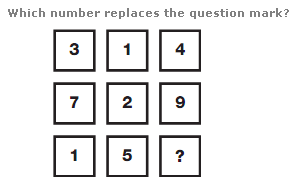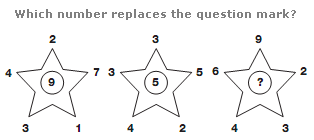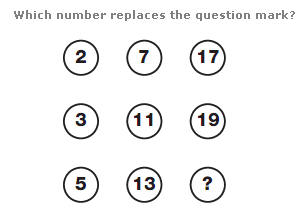# Puzzles - Number puzzles

### Exercise :: Number puzzlesAnswer : 6 Explanation : On each row, add the values of the left hand and central boxes to give the value in the right hand box.Answer : 10 Explanation : In each star diagram, the number in the centre equals the sum of the top three numbers, subtracting the sum of the bottom two numbers.Answer : 23 Explanation : Starting top left, and moving down in columns from left to right, the numbers follow the sequence of Prime Numbers from 2 to 23.